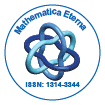# Mathematica EternaOpen Access

ISSN: 1314-3344

## Abstract

### Ruin Probability with Investment Returns and Dependent Structures

Fenglong Guo and Dingcheng Wang

This paper investigates ruin probabilities in a discrete-time risk model, where the premiums are modelled by a Markov chain, while the claims and interest rates follow two first-order autoregressive processes. Recursive and integral equations are given for ruin probabilities in the risk model. Inequalities for ruin probability are derived by recursive techniques

Top